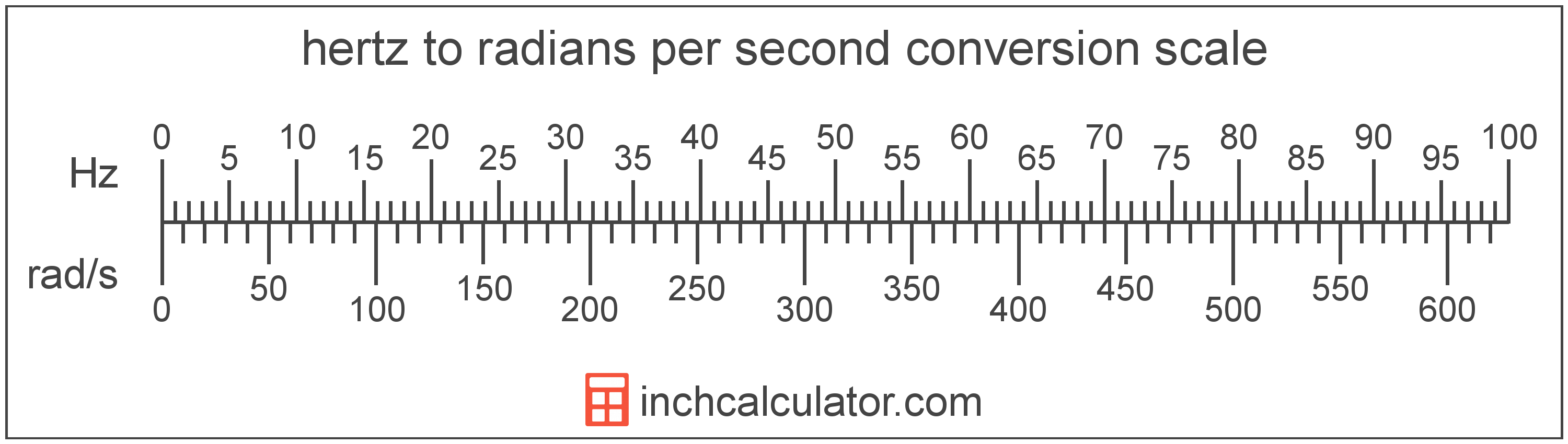# Radians per Second to Hertz Converter

Enter the frequency in radians per second below to get the value converted to hertz.

Results in Hertz:Do you want to convert hertz to radians per second?

## How to Convert Radians per Second to Hertz

To convert a measurement in radians per second to a measurement in hertz, multiply the frequency by the following conversion ratio: 0.159155 hertz/radian per second.

Since one radian per second is equal to 0.159155 hertz, you can use this simple formula to convert:

hertz = radians per second × 0.159155

The frequency in hertz is equal to the frequency in radians per second multiplied by 0.159155.

For example, here's how to convert 5 radians per second to hertz using the formula above.
hertz = (5 rad/s × 0.159155) = 0.795775 Hz### How Many Hertz Are in One Radian per Second?

There are 0.159155 hertz in one radian per second, which is why we use this value in the formula above.

The following formula can also be used to convert the angular frequency in radians per second to frequency in hertz:

ƒ = ω /

In other words, the frequency ƒ in hertz is equal to the angular frequency ω in radians per second divided by 2 times pi.

## What Are Radians per Second?

Radians per second are a measure of angular frequency, or rotational speed, equal to the change in the angular orientation of an object over one second.

In the expressions of units, the slash, or solidus (/), is used to express a change in one or more units relative to a change in one or more other units. For example, rad/s is expressing a change in angle relative to a change in time.

## What is a Hertz?

One hertz is formally defined as the frequency of one cycle per second.

The hertz is the SI derived unit for frequency in the metric system. Hertz can be abbreviated as Hz; for example, 1 hertz can be written as 1 Hz.

Frequency in hertz can be expressed using the formula:

Hz = Cycles / Time in Seconds

## Radian per Second to Hertz Conversion Table

Table showing various radian per second measurements converted to hertz.